>

#### Chapter 20 Surface Area and Volume of a Right Circular Cone R.D. Sharma Solutions for Class 9th MCQ's

Mark the correct alternative in each of the following:

1. The number of surfaces of a cone has, is
(a) 1
(b) 2
(c) 3
(d) 4

Solution

The surfaces or faces that a cone has are :
(1) Base
(2) Slanted Surface
So, the number of surfaces that a cone has is 2.
Hence the correct choice is (b).

2. The area of the curved surface of a cone of radius 2r and slant height l/2, is
(a) πrl
(b) 2πrl
(c) 1/2 πrl
(d) π(r+l)r

Solution

The formula of the curved surface area of a cone with base radius ‘r’ and slant height ‘l’ is given as
Curved Surface Area = πrl
Here the base radius is given as ‘l/2’ and the slant height is given as ‘’
Substituting these values in the above equation we have
Curved Surface Area = (π)(2)(r)(l)/2
= πrl
Hence the correct choice is (a).

3.The total surface area of a cone of radius r/2 and length 2l, is
(a) 2πr (1+r)
(b) πr(1+r/4)
(c) πr (1+r)
(d) 2πrl

Solution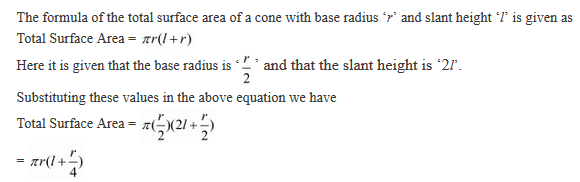4. A solid cylinder is melted and cast into a cone of same radius. The heights of the cone and cylinder are in the ratio
(a) 9 : 1
(b) 1 : 9
(c) 3 : 1
(d) 1 : 3

Solution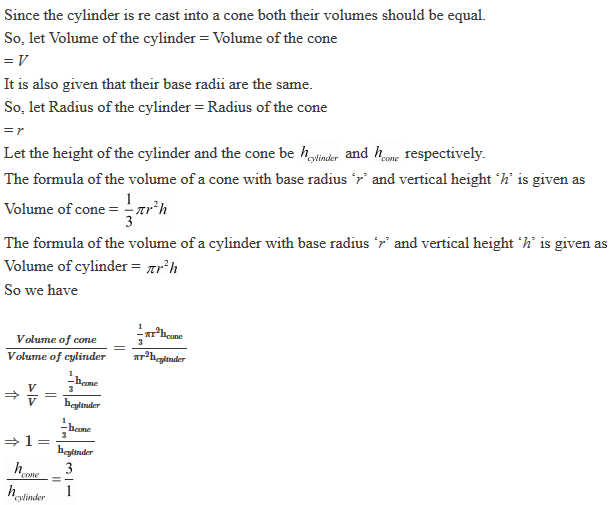5. The slant height of a cone is increased by 10%. If the radius remains the same, the curved surface area is increased by
(a) 10 %
(b) 12.1 %
(c) 20 %
(d) 21 %

Solution

The formula of the curved surface area of a cone with base radius ‘r’ and slant height ‘l’ is given as
Curved Surface Area = πrl
Now, it is said that the slant height has increased by 10%. So the new slant height is ‘1.1l
So, now
New Curved Surface Area = 1.1πrl
We see that the percentage increase of the Curved Surface Area is 10%
Hence the correct option is (a).

6. The height of a solid cone is 12 cm and the area of the circular base is 64πcm2. A plane parallel to the base of the cone cuts through the cone 9 cm above the vertex of the cone, the areas of the base of the new cone so formed is
(a) 9π cm2
(b) 16π cm2
(c) 25π cm2
(d) 36π cm2

Solution

If a cone is cut into two parts by a plane parallel to the base, the portion that contains the base is called the frustum of a cone7. If the radius of the base of a right circular cone is 3r and its height is equal to the radius of the base, then its volume is
(a) 1/3πr3
(b) 2/3πr3
(c) 3πr3
(d) 9πr3

Solution8. If the volume of two cones are in the ratio 1 : 4 and their diameters are in the ratio 4 : 5, then the ratio of their heights, is

(a) 1 : 5
(b) 5 : 4
(c) 5 : 16
(d) 25 : 64

Solution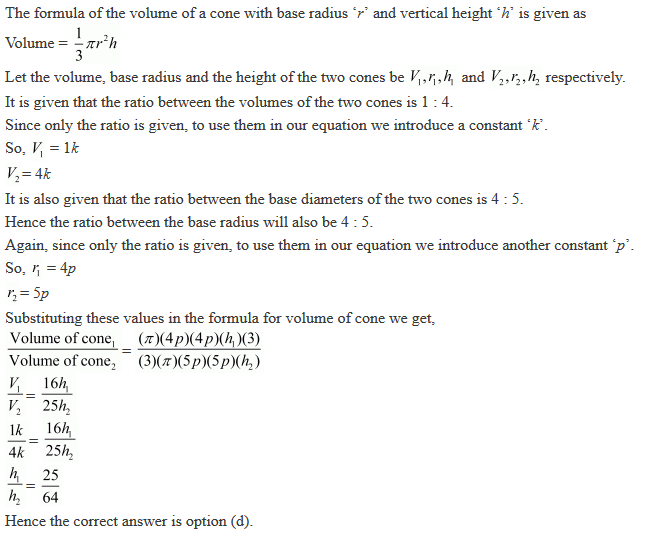9. The curved surface area of one cone is twice that of the other while the slant height of the latter is twice that of the former. The ratio of their radii is
(a) 2 : 1
(b) 4 : 1
(c) 8 : 1
(d) 1 : 1

Solution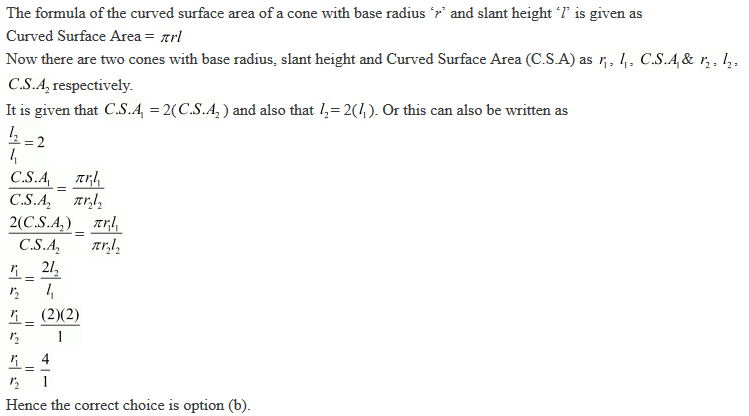10. If the height and radius of a cone of volume V are doubled, then the volume of the cone, is
(a) 3 V
(b) 4 V
(c) 6 V
(d) 8 V

Solution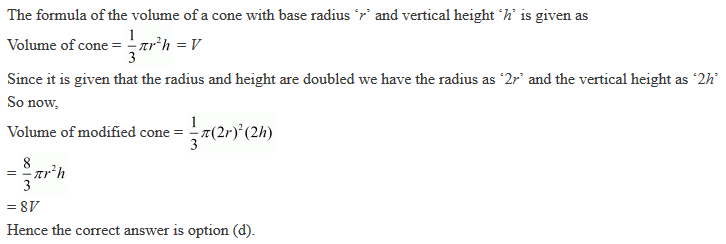11. The ratio of the volume of a right circular cylinder and a right circular cone of the same base and height, is
(a) 1 : 3
(b) 3 : 1
(c) 4 : 3
(d) 3 : 4

Solution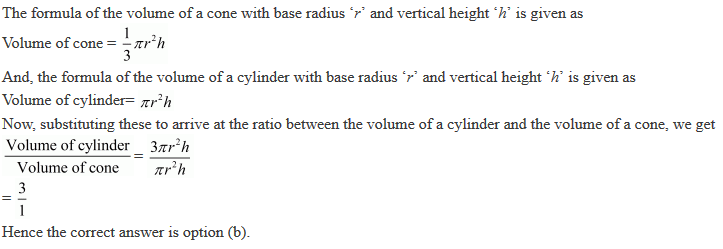12. A right circular cylinder and a right circular cone have the same radius and the same volume. The ratio of the height of the cylinder to that of the cone is
(a) 3 : 5
(b) 2 : 5
(c) 3 : 1
(d) 1 : 3

Solution13. If the base radius and the height of a right circular cone are increased by 20%, then the percentage increase in volume is approximately
(a) 60
(b) 68
(c) 73
(d) 78

Solution14. The diameters of two cones are equal. If their slant heights are in the ratio 5 : 4, the ratio of their curved surface areas, is
(a) 4 : 5
(b) 25 : 16
(c) 16 : 25
(d) 5 : 4

Solution15. If h, S and V denote respectively  the height, curved surface area and volume of a right circular cone, then 3πVh3  − S2h2 + 9V2 is equal to
(a) 8
(b) 0
(c) 4π
(d) 32π2

Solution

Here we are asked to find the value for a given specific equation which is in terms of V, h and S representing the volume, vertical height and the Curved Surface Area of a cone.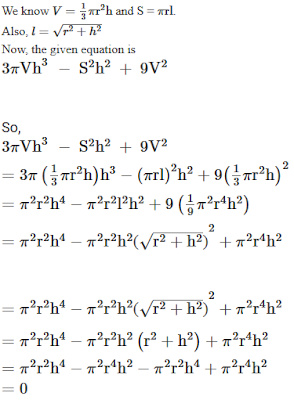16. If a cone is cut into two parts by a horizontal plane passing through the mid-point of its axis, the axis, the ratio of the volumes of upper and lower part is
(a) 1 : 2
(b) 2 : 1
(c) 1: 7
(d) 1 : 8

Solution

If a cone is cut into two parts by a plane parallel to the base, the portion that contains the base is called the frustum of a cone .17. If the heights of two cones are in the ratio of 1 : 4 and the radii of their bases are in the ratio 4 : 1, then the ratio of their volumes is
(a) 1 : 2
(b) 2 : 3
(c) 3 : 4
(d) 4 : 1

Solution